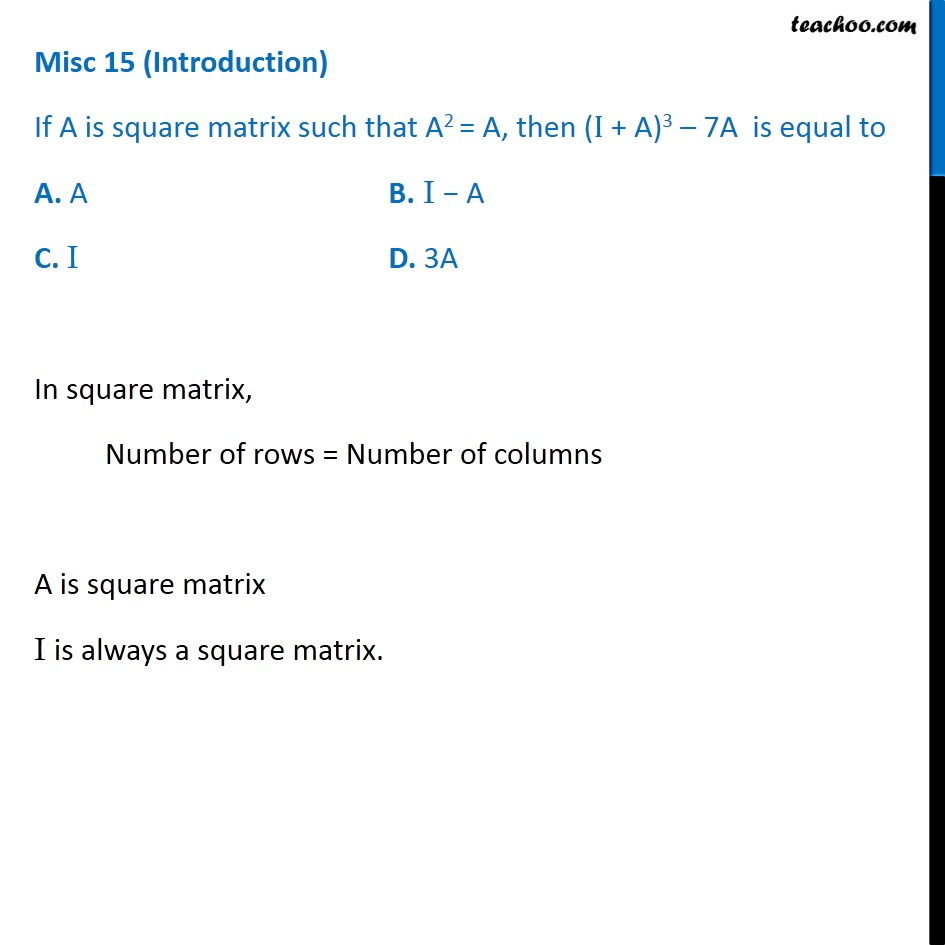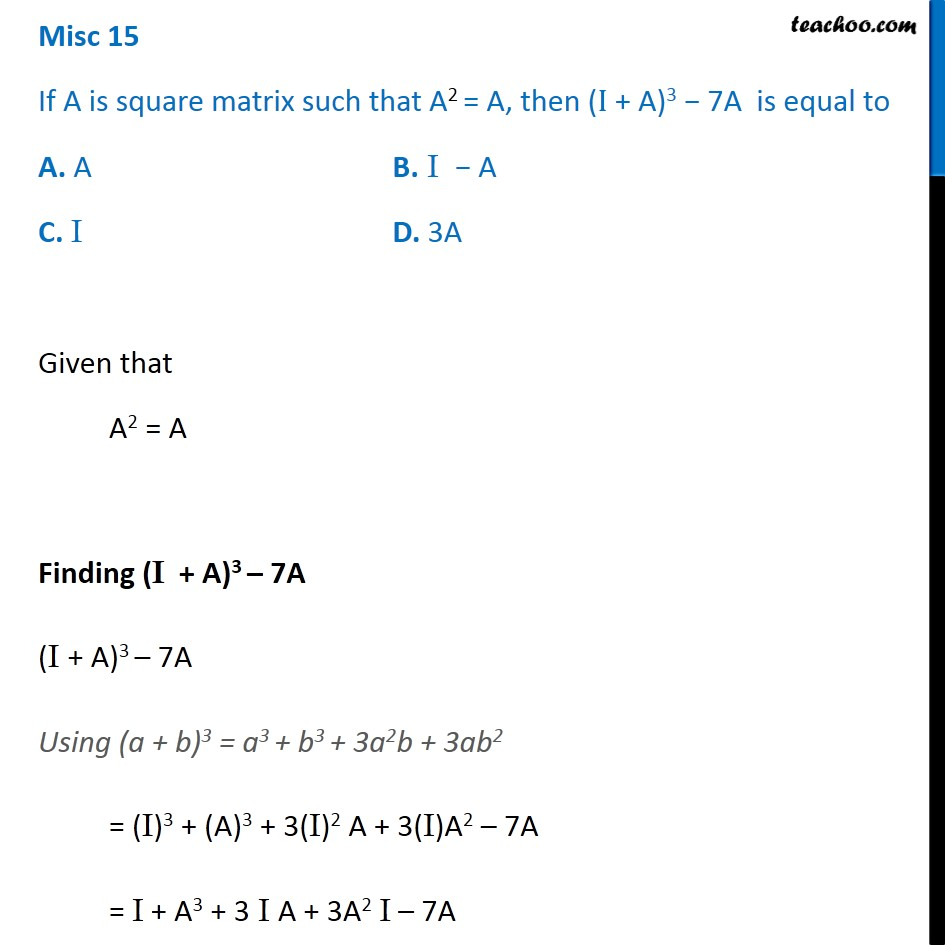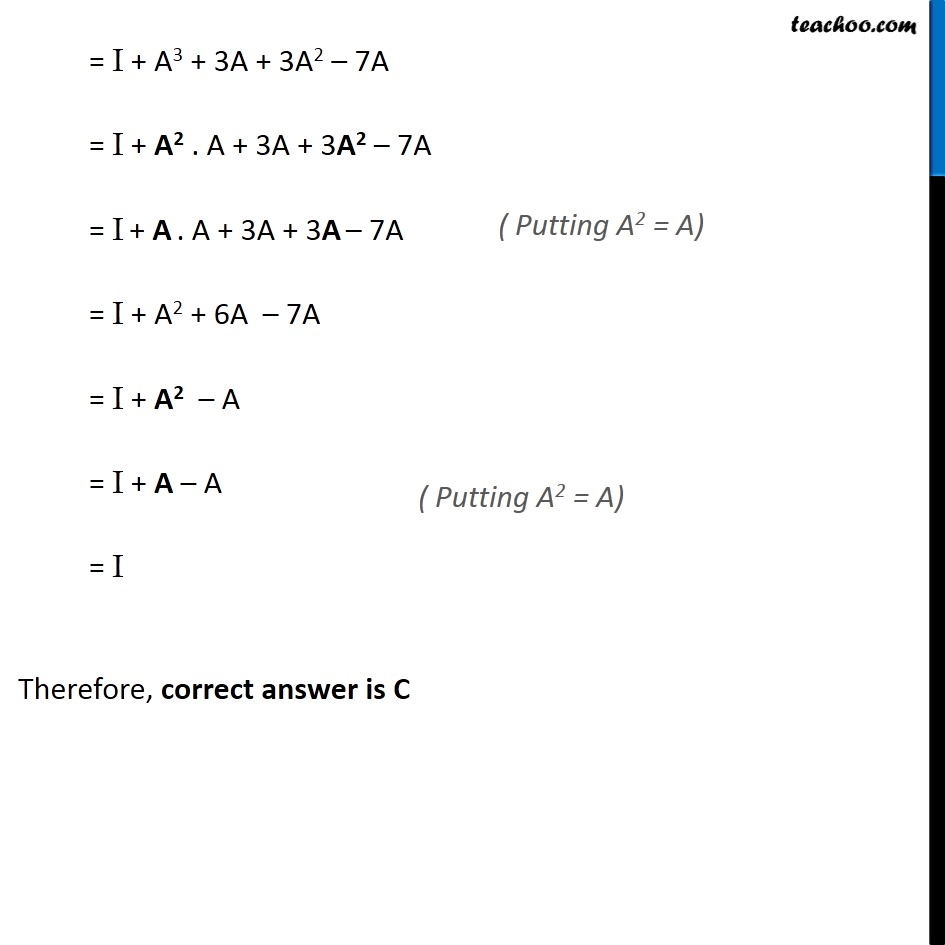1. Chapter 3 Class 12 Matrices (Term 1)
2. Concept wise
3. Solving Equation

Transcript

Misc 15 (Introduction) If A is square matrix such that A2 = A, then (I + A)3 – 7A is equal to A. A B. I − A C. I D. 3A In square matrix, Number of rows = Number of columns A is square matrix I is always a square matrix. Misc 15 If A is square matrix such that A2 = A, then (I + A)3 − 7A is equal to A. A B. I − A C. I D. 3A Given that A2 = A Finding (I + A)3 – 7A (I + A)3 – 7A Using (a + b)3 = a3 + b3 + 3a2b + 3ab2 = (I)3 + (A)3 + 3(I)2 A + 3(I)A2 – 7A = I + A3 + 3 I A + 3A2 I – 7A = I + A3 + 3A + 3A2 – 7A = I + A2 . A + 3A + 3A2 – 7A = I + A . A + 3A + 3A – 7A = I + A2 + 6A – 7A = I + A2 – A = I + A – A = I Therefore, correct answer is C ( Putting A2 = A) ( Putting A2 = A)

Solving Equation

Chapter 3 Class 12 Matrices (Term 1)
Concept wise